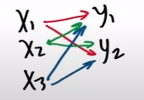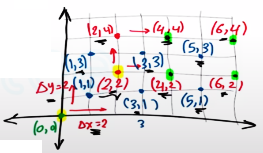Need Help?

Subscribe to Calculus C

###### \${selected_topic_name}
• Notes
• Comments & Questions

(a) Estimate the volume of the solid that lies below the surface
$z-x y$ and above the rectangle
$R-\{(x, y) | 0<x<6,0 \leq y<4\}$

Use a Riemann sum with $m-3, n-2,$ and take the
sample point to be the upper right corner of each square.
(b) Use the Midpoint Rule to estimate the volume of the solid
in part (a).

(a) Rieman Sum

$V=\sum_{i=1}^{m=3} \sum_{j=1}^{n=2} f\left(x_{i}^{*}, y_{j}^{*}\right) D A$

$\Delta A=\Delta x \Delta y=4$

$D A=\left(\frac{6-0}{3}\right)\left(\frac{4 - 0}{2}\right)=4$

$n=2, m=3$
$2 \times 3=6$$f\left(x_{1}^{*}, y_{1}^{*}\right)$
$f\left(x_{1}^{*}, y_{2}^{*}\right)$
$f\left(x_{2}^{*}, y_{1}^{*}\right)$
$f\left(x_{2}^{*}, y_{2}^{*}\right)$

$f\left(x_{3}^{*}, y_{1}^{*}\right)$
$f\left(x_{3}^{*}, y_{2}^{*}\right)$

$V=\Delta A$

$x_{i}^{*}=a+\Delta x i$

$x_{1}^{*}=0+2 * 1=2$

$x_{2}^{*}=0+2 * 2=4$

$x_{3}^{*}=0+2 * 3=6$

$y_{j}^{*}=a+\Delta y j$

$y_{1}^{*}=0+2 * 1=2$
$y_{2}^{*}=0+2 * 2=4$

$f \left(x^{*}, y^{*}\right)=x^{*} y^{*}=x_{1}^{*} y_{1}^{*}$

$f\left(x_{1}^{*}, y_{1}^{*}\right)=4$
$f\left(x_{1}^{*}, y_{2}^{*}\right)=8$
$f\left(x_{2}^{*}, y_{1}^{*}\right)=8$
$f\left(x_{2}^{*}, y_{2}^{*}\right)=16$

$f\left(x_{3}^{*}, y_{1}^{*}\right)=12$
$f\left(x_{3}^{*}, y_{2}^{*}\right)=24$

$V=[4+8+8+16+12+24] 4=288$

$\left(a_{x}, a_{y}\right)=(0,0),\Delta x=2 , \Delta y=2$

$x=3 \quad n=2$

$n * m=2 * 3=6$

$f(x, y)=x y=4$

$v=[4+8+8+16+12+24] 4=288$

(b) Midpoint

$V=[1 * 1+1 * 3+3 * 3+3 * 1+5 * 1+5 * 3] 4$

$V=144$No comments yet